Daniel Morales_

Maker - Data Scientist - Ruby on Rails Fullstack Developer

@daniel_moralesp

2020-12-18 18:00:24 UTC

## Creating the Whole Machine Learning Pipeline with PyCaret

How to Create a Machine Learning Pipeline with PyCaret

This tutorial covers the entire ML process, from data ingestion, pre-processing, model training, hyper-parameter fitting, predicting and storing the model for later use.

We will complete all these steps in less than 10 commands that are naturally constructed and very intuitive to remember, such as

```create_model(),
tune_model(),
compare_models()
plot_model()
evaluate_model()
predict_model()```
Let’s see the whole picture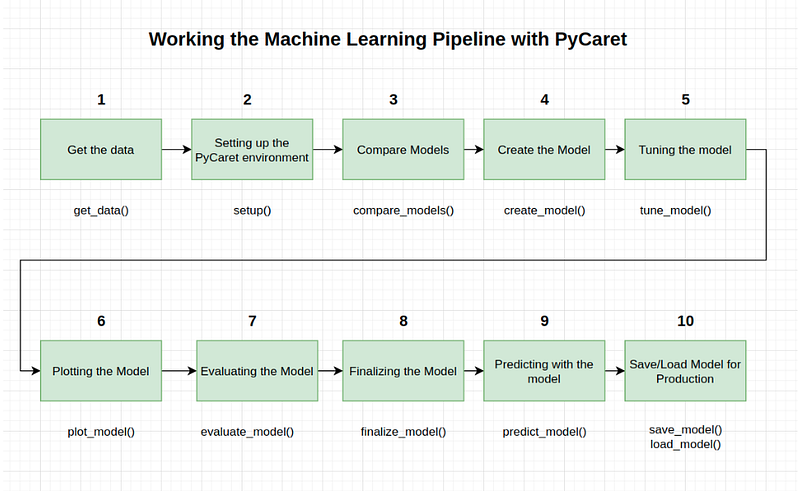Image by Author

Recreating the entire experiment without PyCaret requires more than 100 lines of code in most libraries. The library also allows you to do more advanced things, such as advanced pre-processing, ensembling, generalized stacking, and other techniques that allow you to fully customize the ML pipeline and are a must for any data scientist.

PyCaret is an open source, low-level library for ML with Python that allows you to go from preparing your data to deploying your model in minutes. Allows scientists and data analysts to perform iterative data science experiments from start to finish efficiently and allows them to reach conclusions faster because much less time is spent on programming. This library is very similar to Caret de R, but implemented in python

When working on a data science project, it usually takes a long time to understand the data (EDA and feature engineering). So, what if we could cut the time we spend on the modeling part of the project in half?

Let’s see how

First we need this pre-requisites

• Python 3.6 or later
• PyCaret 2.0 or later
Here you can find the library docs and others.

Also, you can follow this notebook with the code.

First of all, please run this command: !pip3 install pycaret

For Google Colab users: If you are running this notebook in Google Colab, run the following code at the top of your notebook to display interactive images

```from pycaret.utils import enable_colab
enable_colab()```
Pycaret Modules

Pycaret is divided according to the task we want to perform, and has different modules, which represent each type of learning (supervised or unsupervised). For this tutorial, we will be working on the supervised learning module with a binary classification algorithm.

Classification Module

The PyCaret classification module (pycaret.classification) is a supervised machine learning module used to classify elements into a binary group based on various techniques and algorithms. Some common uses of classification problems include predicting client default (yes or no), client abandonment (client will leave or stay), disease encountered (positive or negative) and so on.

The PyCaret classification module can be used for binary or multi-class classification problems. It has more than 18 algorithms and 14 plots for analyzing model performance. Whether it’s hyper-parameter tuning, ensembling or advanced techniques such as stacking, PyCaret’s classification module has it all.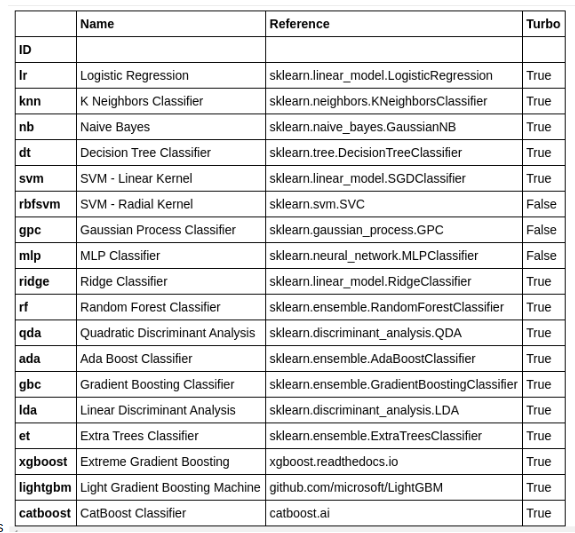Image by Author

For this tutorial we will use an UCI data set called Default of Credit Card Clients Dataset. This data set contains information about default payments, demographics, credit data, payment history and billing statements of credit card customers in Taiwan from April 2005 to September 2005. There are 24,000 samples and 25 characteristics.

In :

`import pandas as pd`
In :

`df = pd.read_csv('datasets/default of credit card clients.csv')`
In :

`df.head()`
Out: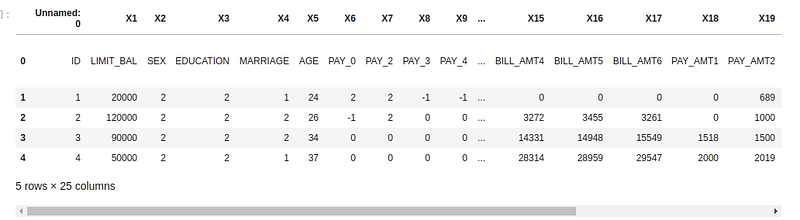Image by Author

1- Get the data

We also have another way to load it. In fact this will be the default way we will be working with in this tutorial. It is directly from the PyCaret datasets, and it is the first method of our Pipeline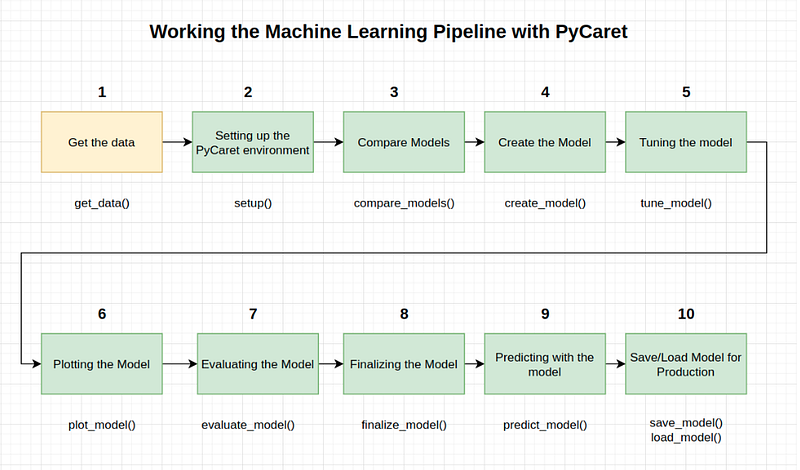Image by Author

```from pycaret.datasets import get_data
dataset = get_data('credit')#check the shape of data
dataset.shape```
In order to demonstrate the predict_model() function on unseen data, a sample of 1200 records from the original dataset has been retained for use in the predictions. This should not be confused with a train/test split, since this particular split is made to simulate a real-life scenario. Another way of thinking about this is that these 1200 records are not available at the time the ML experiment was performed.

In :

```## sample returns a random sample from an axis of the object. That would be 22,800 samples, not 24,000
data = dataset.sample(frac=0.95, random_state=786)```
In :

`data`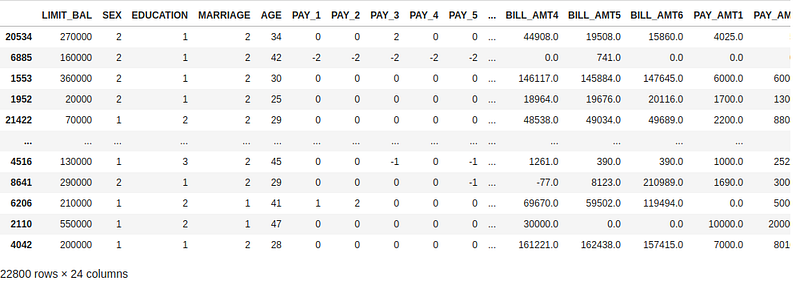Image by Author

```# we remove from the original dataset this random data
data_unseen = dataset.drop(data.index)```
In :

```data_unseen## we reset the index of both datasets
data.reset_index(inplace=True, drop=True)
data_unseen.reset_index(inplace=True, drop=True)
print('Data for Modeling: ' + str(data.shape))
print('Unseen Data For Predictions: ' + str(data_unseen.shape))Data for Modeling: (22800, 24)
Unseen Data For Predictions: (1200, 24)```
Split data

The way we divide our data set is important because there is data that we'll not use during the modeling process, and we'll use at the end to validate our results by simulating real data. The data we use for modeling we sub-divide it in order to evaluate two scenarios, training and testing. Therefore, the following has been done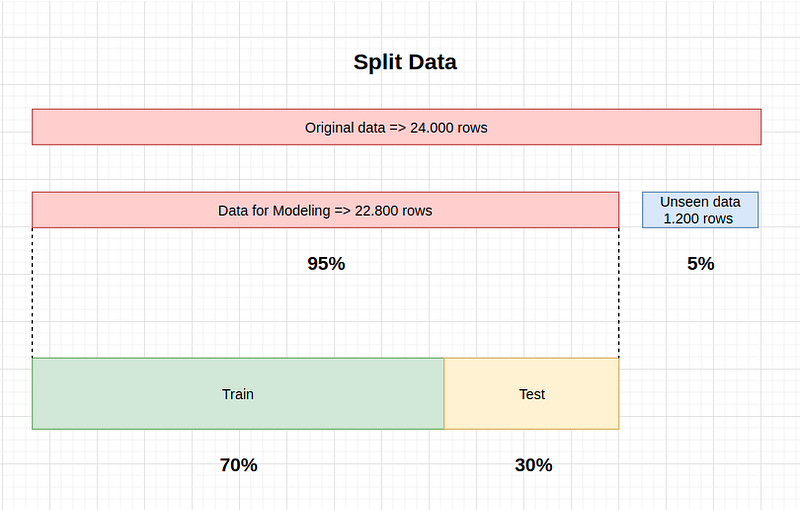Image by Author

Unseen data set (also known as validation data set)

• Is the data sample used to provide an unbiased assessment of a final model.
• The validation data set provides the gold standard used to evaluate the model.
• It is only used once the model is fully trained (using the training and test sets).
• The validation set is generally what is used to evaluate the models of a competition (for example, in many Kaggle or DataSource.ai competitions, the test set is initially released along with the training and test set and the validation set is only released when the competition is about to close, and it is the result of the validation set model that decides the winner).
• Many times the test set is used as the validation set, but it is not a good practice.
• The validation set is generally well healed.
• It contains carefully sampled data covering the various classes that the model would face, when used in the real world.
Training data set

• Training data set: The data sample used to train the model.
• The data set we use to train the model
• The model sees and learns from this data.
Test data set

• Test Data Set: The data sample used to provide an unbiased assessment of a model is matched to the training data set while adjusting the model’s hyperparameters.
• The assessment becomes more biased as the skill in the test data set is incorporated into the model configuration.
• The test set is used to evaluate a given model, but this is for frequent evaluation.
• We, as ML engineers, use this data to fine-tune the hyperparameters of the model.
• Therefore, the model occasionally sees this data, but never “learns” from it.
• We use the results of the test set, and update the higher level hyperparameters
• So the test set impacts a model, but only indirectly.
• The test set is also known as the Development set. This makes sense, since this dataset helps during the “development” stage of the model.
Confusion of terms

• There is a tendency to mix up the name of test and validation.
• Depending on the tutorial, the source, the book, the video or the teacher/mentor the terms are changed, the important thing is to keep the concept.
• In our case we already separated the validation set at the beginning (1,200 samples of data_unseen)
2- Setting up the PyCaret environment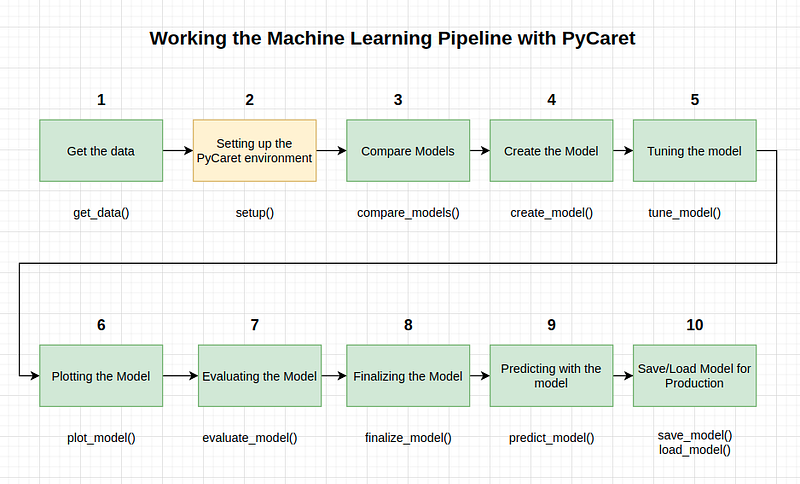Image by Author

Now let’s set up the Pycaret environment. The setup() function initializes the environment in pycaret and creates the transformation pipeline to prepare the data for modeling and deployment. setup() must be called before executing any other function in pycaret. It takes two mandatory parameters: a pandas dataframe and the name of the target column. Most of this part of the configuration is done automatically, but some parameters can be set manually. For example:

• The default division ratio is 70:30 (as we see in above paragraph), but can be changed with "train_size".
• K-fold cross-validation is set to 10 by default
• “session_id" is our classic "random_state"
In :

```## setting up the environment
from pycaret.classification import *```
Note: After you run the following command you must press enter to finish the process. We will explain how they do it. The setup process may take some time to complete.

In :

`model_setup = setup(data=data, target='default', session_id=123)`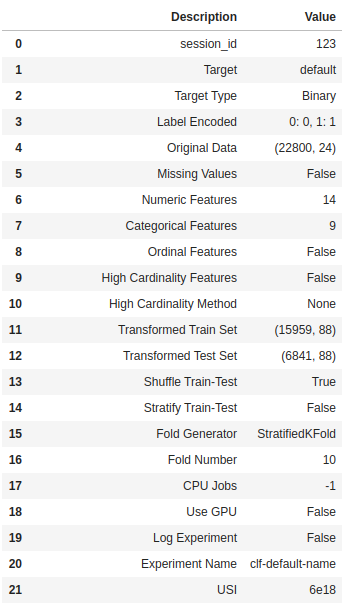Image by Author

When you run setup(), PyCaret's inference algorithm will automatically deduce the data types of all features based on certain properties. The data type must be inferred correctly but this is not always the case. To take this into account, PyCaret displays a table containing the features and their inferred data types after setup() is executed. If all data types are correctly identified, you can press enter to continue or exit to end the experiment. We press enter, and should come out the same output as we got above.

Ensuring that the data types are correct is critical in PyCaret, as it automatically performs some pre-processing tasks that are essential to any ML experiment. These tasks are performed differently for each type of data, which means that it is very important that they are correctly configured.

We could overwrite the type of data inferred from PyCaret using the numeric_features and categorical_features parameters in setup(). Once the setup has been successfully executed, the information grid containing several important pieces of information is printed. Most of the information is related to the pre-processing pipeline that is built when you run setup()

Most of these features are out of scope for the purposes of this tutorial, however, some important things to keep in mind at this stage include

• session_id : A pseduo-random number distributed as a seed in all functions for later reproducibility.
• Target type : Binary or Multiclass. The target type is automatically detected and displayed.
• Label encoded: When the Target variable is of type string (i.e. ‘Yes’ or ‘No’) instead of 1 or 0, it automatically codes the label at 1 and 0 and shows the mapping (0 : No, 1 : Yes) as reference
• Original data : Displays the original form of the data set. In this experiment (22800, 24) ==> Remember: "Seeing data"
• Missing values : When there are missing values in the original data this will be shown as True
• Numerical features : The number of features inferred as numerical.
• Categorical features : The number of features inferred as categorical
• Transformed train sets: Note that the original form of (22800, 24) is transformed into (15959, 91) for the transformed train set and the number of features has increased from 24 to 91 due to the categorical coding
• Transformed test set: There are 6,841 samples in the test set. This split is based on the default value of 70/30 which can be changed using the train_size parameter in the configuration.
Note how some tasks that are imperative to perform the modeling are handled automatically, such as imputation of missing values (in this case there are no missing values in the training data, but we still need imputers for the unseen data), categorical encoding, etc.

Most of the setup() parameters are optional and are used to customize the preprocessing pipeline.

3- Compare Models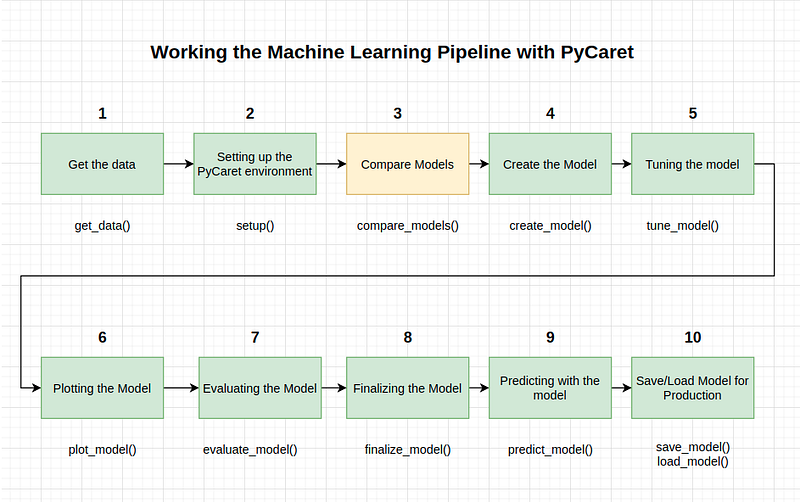Image by Author

In order to understand how PyCaret compares the models and the next steps in the pipeline, it is necessary to understand the concept of N-Fold Coss-Validation.

N-Fold Coss-Validation

Calculating how much of your data should be divided into your test set is a delicate question. If your training set is too small, your algorithm may not have enough data to learn effectively. On the other hand, if your test set is too small, then your accuracy, precision, recall and F1 score could have a large variation.

You may be very lucky or very unlucky! In general, putting 70% of your data in the training set and 30% of your data in the test set is a good starting point. Sometimes your data set is so small that dividing it 70/30 will result in a large amount of variance.

One solution to this is to perform N-Fold cross-validation. The central idea here is that we are going to do this whole process N times and then average the accuracy. For example, in a 10 times cross validation, we will make the test set the first 10% of the data and calculate the accuracy, precision, recall and F1 score.

Then, we will make the cross-validation establish the second 10% of the data and we will calculate these statistics again. We can do this process 10 times, and each time the test set will be a different piece of data. Then we average all the accuracies, and we will have a better idea of how our model works on average.

Note: Validation Set (yellow here) is the Test Set in our case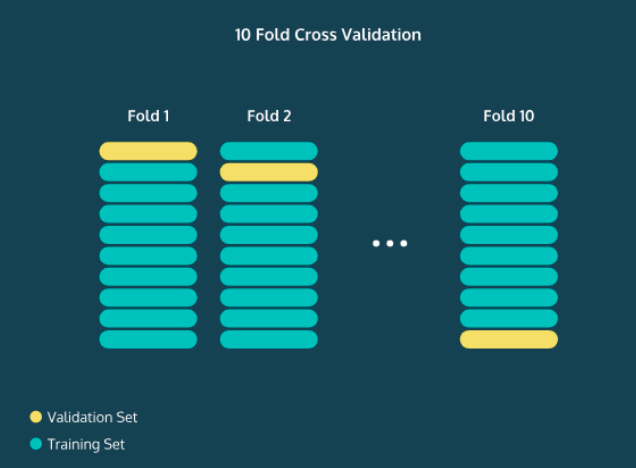Image by Author

Understanding the accuracy of your model is invaluable because you can start adjusting the parameters of your model to increase its performance. For example, in the K-Nearest Neighbors algorithm, you can see what happens to the accuracy as you increase or decrease K. Once you are satisfied with the performance of your model, it's time to enter the validation set. This is the part of your data that you split at the beginning of his experiment (unseen_data in our case).

It is supposed to be a substitute for the real-world data that you are really interested in sorting out. It works very similar to the test set, except that you never touched this data while building or refining your model. By finding the precision metrics, you get a good understanding of how well your algorithm will perform in the real world.

Comparing all models

Comparing all models to evaluate performance is the recommended starting point for modeling once the PyCaret setup() is completed (unless you know exactly what type of model is needed, which is often not the case), this function trains all models in the model library and scores them using a stratified cross-validation for the evaluation of the metrics.

The output prints a score grid that shows the average of the Accuracy, AUC, Recall, Precision, F1, Kappa, and MCC across the folds (10 by default) along with the training times. Let's do it!

In :

`best_model = compare_models()`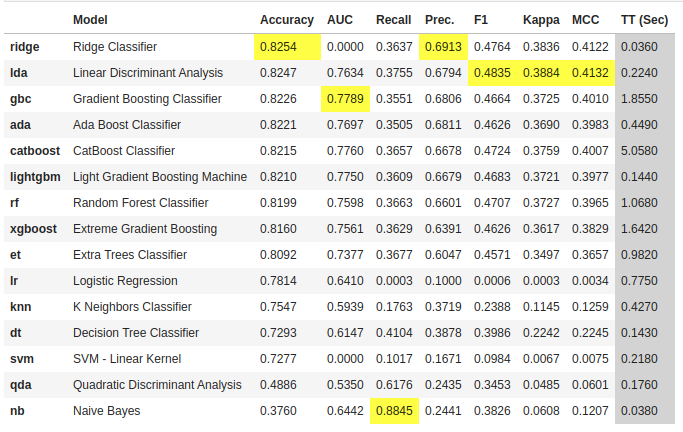Image by Author

The compare_models() function allows you to compare many models at once. This is one of the great advantages of using PyCaret. In one line, you have a comparison table between many models. Two simple words of code (not even one line) have trained and evaluated more than 15 models using the N-Fold cross-validation.

The above printed table highlights the highest performance metrics for comparison purposes only. The default table is sorted using “Accuracy” (highest to lowest) which can be changed by passing a parameter. For example, compare_models(sort = 'Recall') will sort the grid by Recall instead of Accuracy.

If you want to change the Fold parameter from the default value of 10 to a different value, you can use the fold parameter. For example compare_models(fold = 5) will compare all models in a 5-fold cross-validation. Reducing the number of folds will improve the training time.

By default, compare_models returns the best performing model based on the default sort order, but it can be used to return a list of the top N models using the n_select parameter. In addition, it returns some metrics such as accuracy, AUC and F1. Another cool thing is how the library automatically highlights the best results. Once you choose your model, you can create it and then refine it. Let's go with other methods.

In :

```print(best_model)RidgeClassifier(alpha=1.0, class_weight=None, copy_X=True, fit_intercept=True,
max_iter=None, normalize=False, random_state=123, solver='auto',
tol=0.001)```
4- Create the Model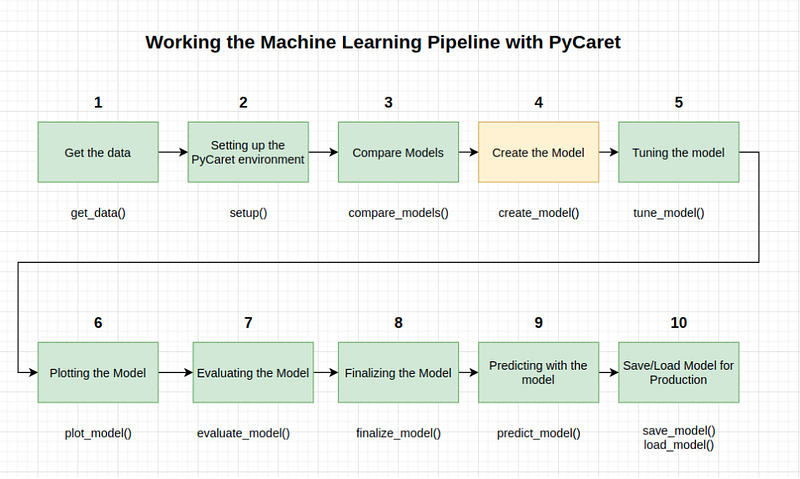Image by Author

create_model is the most granular function in PyCaret and is often the basis for most of PyCaret's functionality. As its name indicates, this function trains and evaluates a model using a cross-validation that can be set with the parameter fold. The output prints a scoring table showing by Fold the Precision, AUC, Recall, F1, Kappa and MCC.

For the rest of this tutorial, we will work with the following models as our candidate models. The selections are for illustrative purposes only and do not necessarily mean that they are the best performers or ideal for this type of data

• Decision Tree Classifier (‘dt’)
• K Neighbors Classifier (‘knn’)
• Random Forest Classifier (‘rf’)
There are 18 classifiers available in the PyCaret model library. To see a list of all classifiers, check the documentation or use the models() function to view the library.

In :

`models()`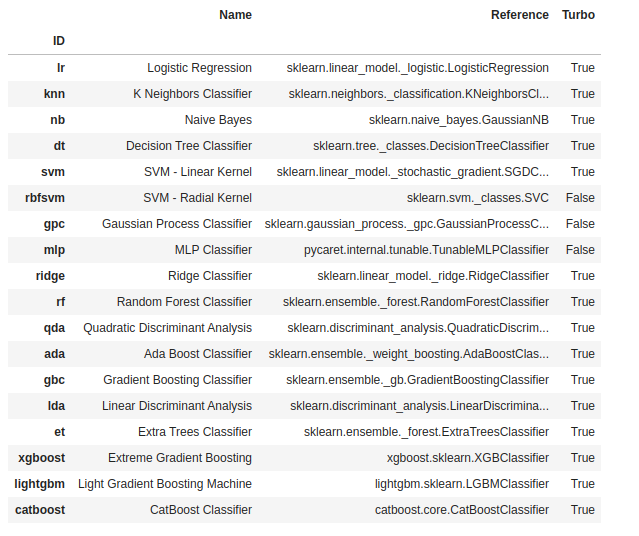Image by Author

`dt = create_model('dt')`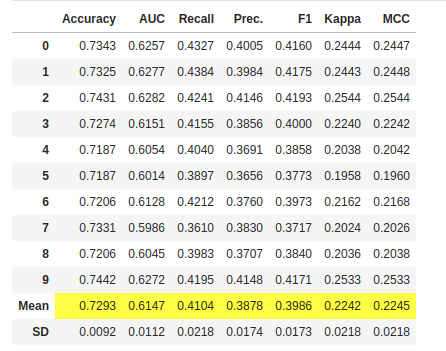Image by Author

```#trained model object is stored in the variable 'dt'.
print(dt)DecisionTreeClassifier(ccp_alpha=0.0, class_weight=None, criterion='gini',
max_depth=None, max_features=None, max_leaf_nodes=None,
min_impurity_decrease=0.0, min_impurity_split=None,
min_samples_leaf=1, min_samples_split=2,
min_weight_fraction_leaf=0.0, presort='deprecated',
random_state=123, splitter='best')```
In :

`knn = create_model('knn')`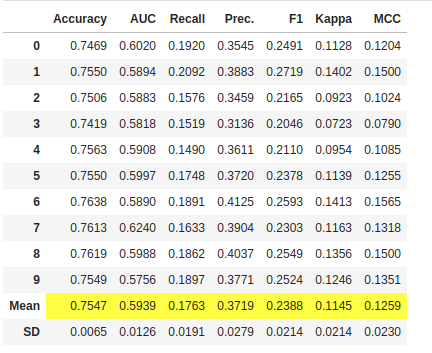Image by Author

```print(knn)KNeighborsClassifier(algorithm='auto', leaf_size=30, metric='minkowski',
metric_params=None, n_jobs=-1, n_neighbors=5, p=2,
weights='uniform')```
In :

`rf = create_model('rf')`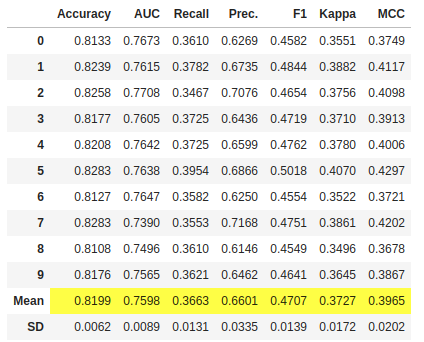Image by Author

```print(rf)RandomForestClassifier(bootstrap=True, ccp_alpha=0.0, class_weight=None,
criterion='gini', max_depth=None, max_features='auto',
max_leaf_nodes=None, max_samples=None,
min_impurity_decrease=0.0, min_impurity_split=None,
min_samples_leaf=1, min_samples_split=2,
min_weight_fraction_leaf=0.0, n_estimators=100,
n_jobs=-1, oob_score=False, random_state=123, verbose=0,
warm_start=False)```
Note that the average score of all models matches the score printed on compare_models(). This is because the metrics printed in the compare_models() score grid are the average scores of all the folds.

You can also see in each print() of each model the hyperparameters with which they were built. This is very important because it is the basis for improving them. You can see the parameters for RandomForestClassifier

```max_depth=None
max_features='auto'
min_samples_leaf=1
min_samples_split=2
min_weight_fraction_leaf=0.0
n_estimators=100
n_jobs=-1```
5- Tunning the Model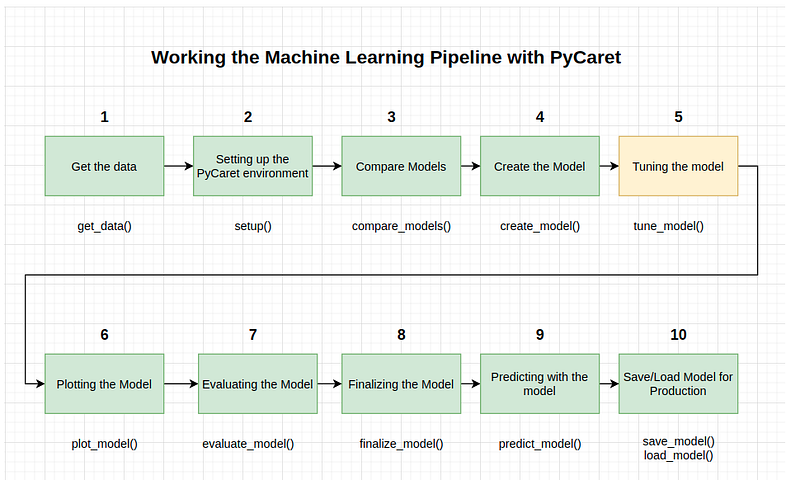Image by Author

When creating a model using the create_model() function the default hyperparameters are used to train the model. To tune the hyperparameters the tune_model() function is used. This function automatically tunes the hyperparameters of a model using the Random Grid Search in a predefined search space.

The output prints a score grid showing the accuracy, AUC, Recall, Precision, F1, Kappa and MCC by Fold for the best model. To use a custom search grid, you can pass the custom_grid parameter in the tune_model function

In :

`tuned_rf = tune_model(rf)`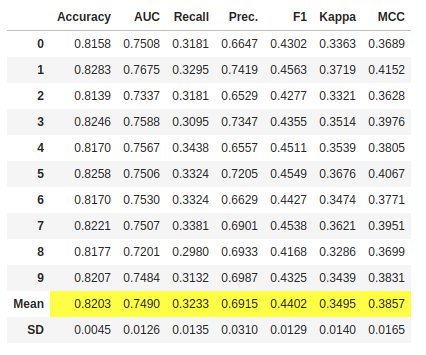Image by Author

If we compare the Accuracy metrics of this refined RandomForestClassifier model with the previous RandomForestClassifier, we see a difference, because it went from an Accuracy of 0.8199 to an Accuracy of 0.8203.

In :

```#tuned model object is stored in the variable 'tuned_dt'.
print(tuned_rf)RandomForestClassifier(bootstrap=False, ccp_alpha=0.0, class_weight={},
criterion='entropy', max_depth=5, max_features=1.0,
max_leaf_nodes=None, max_samples=None,
min_impurity_decrease=0.0002, min_impurity_split=None,
min_samples_leaf=5, min_samples_split=10,
min_weight_fraction_leaf=0.0, n_estimators=150,
n_jobs=-1, oob_score=False, random_state=123, verbose=0,
warm_start=False)```
Let’s compare now the hyperparameters. We had these before.

```max_depth=None
max_features='auto'
min_samples_leaf=1
min_samples_split=2
min_weight_fraction_leaf=0.0
n_estimators=100
n_jobs=-1```
Now these:

```max_depth=5
max_features=1.0
min_samples_leaf=5
min_samples_split=10
min_weight_fraction_leaf=0.0
n_estimators=150
n_jobs=-1```
You can make this same comparisson with knn and dt by yourself and explore the differences in the hyperparameters.

By default, tune_model optimizes Accuracy but this can be changed using the optimize parameter. For example: tune_model(dt, optimize = 'AUC') will look for the hyperparameters of a Decision Tree Classifier that results in the highest AUC instead of Accuracy. For the purposes of this example, we have used Accuracy's default metric only for simplicity.

Generally, when the data set is unbalanced (like the credit data set we are working with) Accuracy is not a good metric to consider. The methodology underlying the selection of the correct metric to evaluate a rating is beyond the scope of this tutorial.

Metrics alone are not the only criteria you should consider when selecting the best model for production. Other factors to consider include training time, standard deviation of k-folds, etc. For now, let’s go ahead and consider the Random Forest Classifier tuned_rf, as our best model for the rest of this tutorial

6- Plotting the Model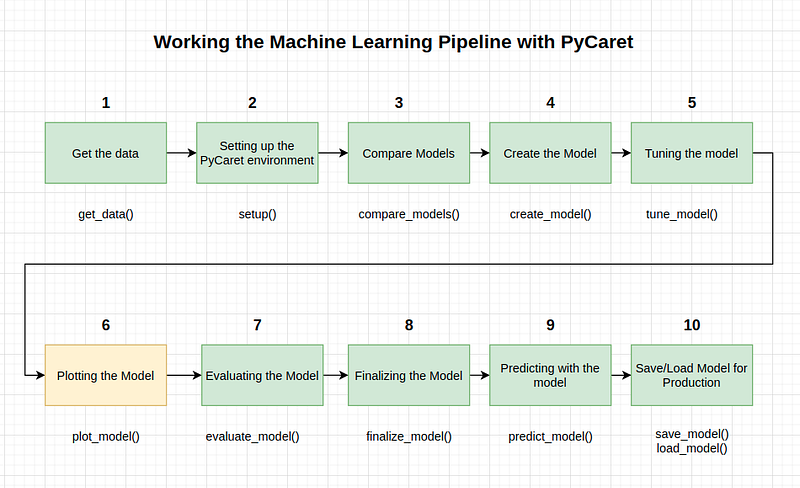Image by Author

Before finalizing the model (Step # 8), the plot_model() function can be used to analyze the performance through different aspects such as AUC, confusion_matrix, decision boundary etc. This function takes a trained model object and returns a graph based on the training/test set.

There are 15 different plots available, please refer to plot_model() documentation for a list of available plots.

In :

`## AUC Plotplot_model(tuned_rf, plot = 'auc')`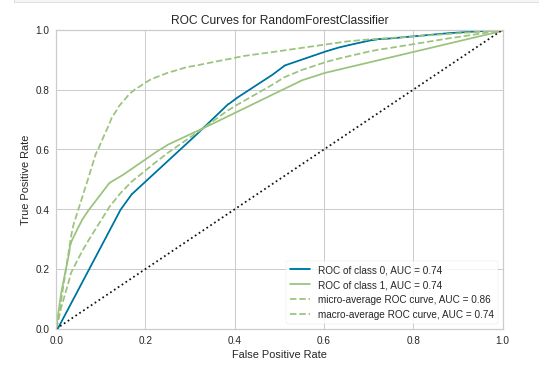Image by Author

```## Precision-recall curve

plot_model(tuned_rf, plot = 'pr')```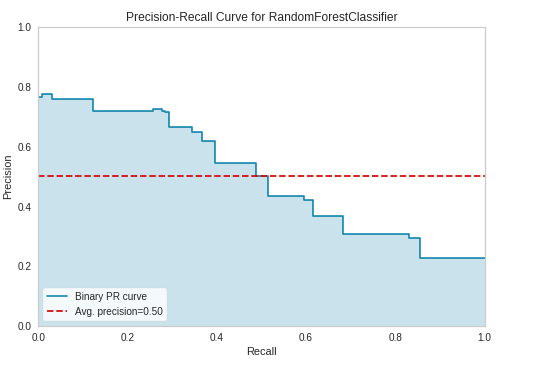Image by Author

```## feature importance

plot_model(tuned_rf, plot='feature')```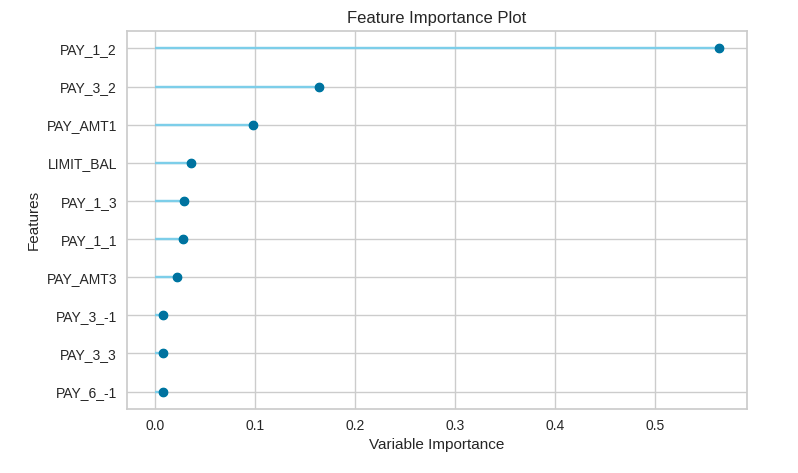Image by Author

```## Consufion matrix

plot_model(tuned_rf, plot = 'confusion_matrix')```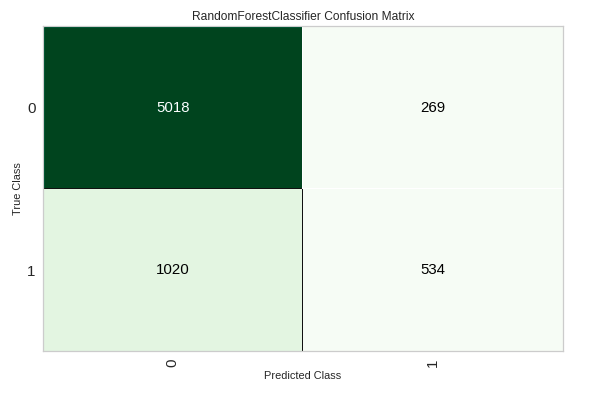Image by Author

7- Evaluating the model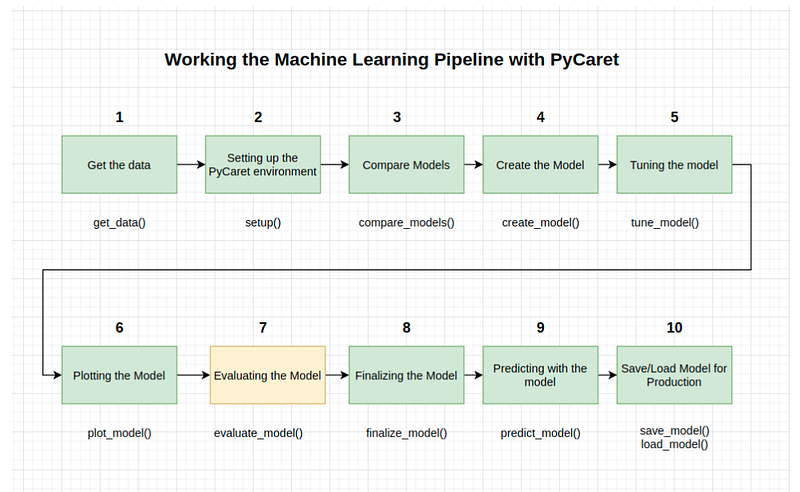Image by Author

Another way to analyze model performance is to use the evaluate_model() function which displays a user interface for all available graphics for a given model. Internally it uses the plot_model() function.

In :

`evaluate_model(tuned_rf)`
8- Finalizing the Model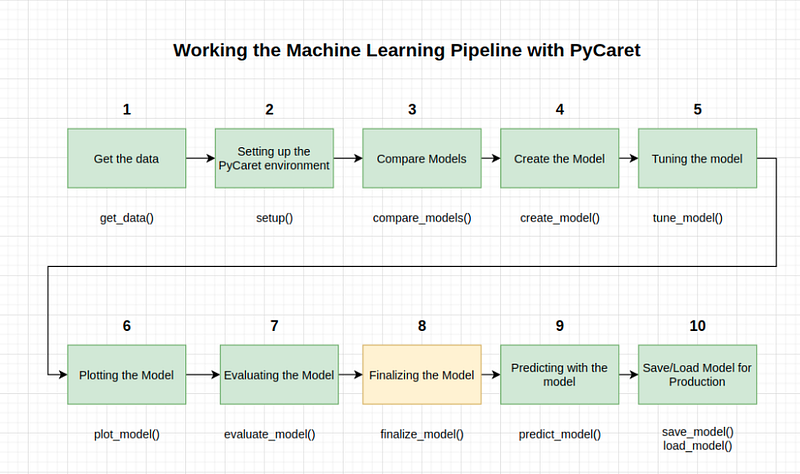Image by Author

The completion of the model is the last step of the experiment. A normal machine learning workflow in PyCaret starts with setup(), followed by comparison of all models using compare_models() and pre-selection of some candidate models (based on the metric of interest) to perform various modeling techniques, such as hyperparameter fitting, assembly, stacking, etc.

This workflow will eventually lead you to the best model to use for making predictions on new and unseen data. The finalize_model() function fits the model to the complete data set, including the test sample (30% in this case). The purpose of this function is to train the model on the complete data set before it is deployed into production. We can execute this method after or before the predict_model(). We're going to execute it after of it.

One last word of caution. Once the model is finalized using finalize_model(), the entire data set, including the test set, is used for training. Therefore, if the model is used to make predictions about the test set after finalize_model() is used, the printed information grid will be misleading since it is trying to make predictions about the same data that was used for the modeling.

To demonstrate this point, we will use final_rf in predict_model() to compare the information grid with the previous.

In :

`final_rf = finalize_model(tuned_rf)`
In :

```#Final Random Forest model parameters for deployment
print(final_rf)RandomForestClassifier(bootstrap=False, ccp_alpha=0.0, class_weight={},
criterion='entropy', max_depth=5, max_features=1.0,
max_leaf_nodes=None, max_samples=None,
min_impurity_decrease=0.0002, min_impurity_split=None,
min_samples_leaf=5, min_samples_split=10,
min_weight_fraction_leaf=0.0, n_estimators=150,
n_jobs=-1, oob_score=False, random_state=123, verbose=0,
warm_start=False)```
9- Predicting with the model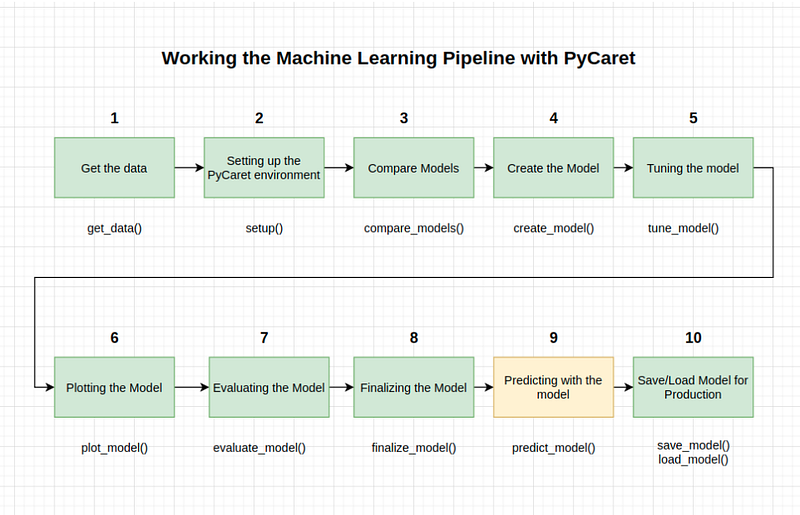Image by Author

Before finalizing the model, it is advisable to perform a final check by predicting the test/hold-out set (data_unseen in our case) and reviewing the evaluation metrics. If you look at the information table, you will see that 30% (6,841 samples) of the data have been separated as training/set samples.

All of the evaluation metrics we have seen above are cross-validated results based on the training set (70%) only. Now, using our final training model stored in the tuned_rf variable we predict against the test sample and evaluate the metrics to see if they are materially different from the CV results

In :

`predict_model(final_rf)`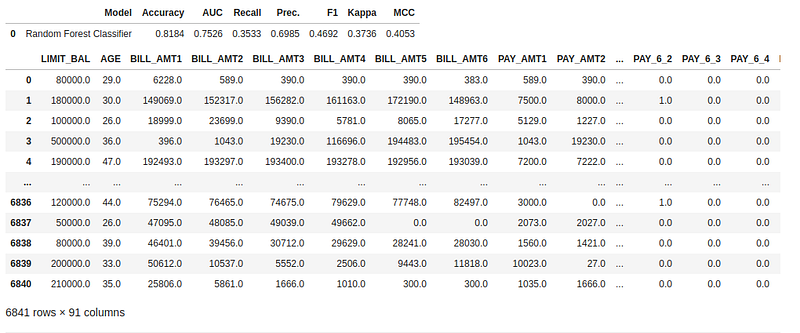Image by Author

The accuracy of the test set is 0.8199 compared to the 0.8203 achieved in the results of the tuned_rf. This is not a significant difference. If there is a large variation between the results of the test set and the training set, this would normally indicate an over-fitting, but it could also be due to several other factors and would require further investigation.

In this case, we will proceed with the completion of the model and the prediction on unseen data (the 5% that we had separated at the beginning and that was never exposed to PyCaret).

(TIP: It is always good to look at the standard deviation of the results of the training set when using create_model().

The predict_model() function is also used to predict about the unseen data set. The only difference is that this time we will pass the parameter data_unseen. data_unseen is the variable created at the beginning of the tutorial and contains 5% (1200 samples) of the original data set that was never exposed to PyCaret.

In :

```unseen_predictions = predict_model(final_rf, data=data_unseen)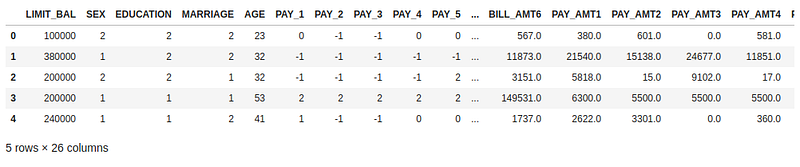Image by Author

Please go to the last column of this previous result, and you will see a new feature called Score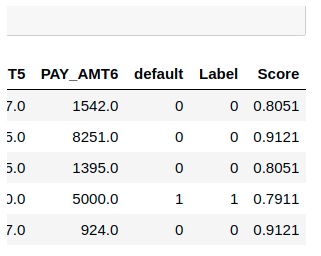Image by Author

Label is the prediction and score is the probability of the prediction. Note that the predicted results are concatenated with the original data set, while all transformations are automatically performed in the background.

We have finished the experiment finalizing the tuned_rf model that now is stored in the final_rf variable. We have also used the model stored in final_rf to predict data_unseen. This brings us to the end of our experiment, but one question remains: What happens when you have more new data to predict? Do you have to go through the whole experiment again? The answer is no, PyCaret's built-in save_model() function allows you to save the model along with all the transformation pipe for later use and is stored in a Pickle in the local environment

(TIP: It’s always good to use the date in the file name when saving models, it’s good for version control)

Let’s see it in the next step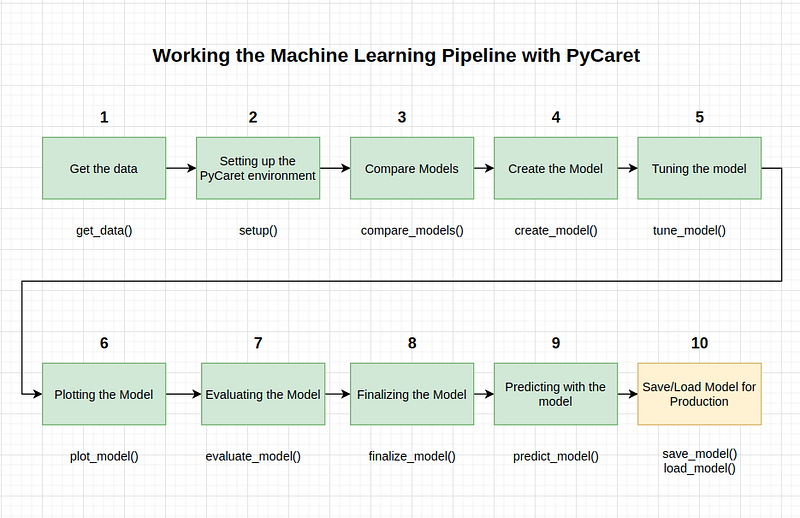Image by Author

Save Model

In :

`save_model(final_rf, 'datasets/Final RF Model 19Nov2020')Transformation Pipeline and Model Succesfully Saved`
Out:

```(Pipeline(memory=None,
steps=[('dtypes',
DataTypes_Auto_infer(categorical_features=[],
display_types=True, features_todrop=[],
id_columns=[],
ml_usecase='classification',
numerical_features=[], target='default',
time_features=[])),
('imputer',
Simple_Imputer(categorical_strategy='not_available',
fill_value_categorical=None,
fill_value_numerical=None,
numeric_stra...
RandomForestClassifier(bootstrap=False, ccp_alpha=0.0,
class_weight={}, criterion='entropy',
max_depth=5, max_features=1.0,
max_leaf_nodes=None, max_samples=None,
min_impurity_decrease=0.0002,
min_impurity_split=None,
min_samples_leaf=5,
min_samples_split=10,
min_weight_fraction_leaf=0.0,
n_estimators=150, n_jobs=-1,
oob_score=False, random_state=123,
verbose=0, warm_start=False)]],
verbose=False),
'datasets/Final RF Model 19Nov2020.pkl')```

To load a model saved at a future date in the same or an alternative environment, we would use PyCaret’s load_model() function and then easily apply the saved model to new unseen data for the prediction

In :

`saved_final_rf = load_model('datasets/Final RF Model 19Nov2020')Transformation Pipeline and Model Successfully Loaded`
Once the model is loaded into the environment, it can simply be used to predict any new data using the same predict_model() function. Next we have applied the loaded model to predict the same data_unseen we used before.

In :

`new_prediction = predict_model(saved_final_rf, data=data_unseen)`
In :

`new_prediction.head()`
Out: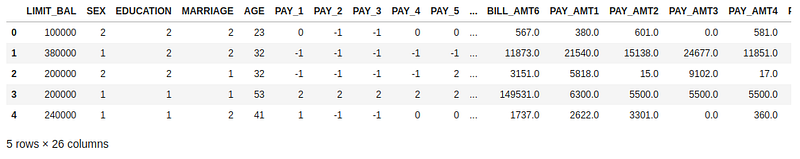Image by Author

```from pycaret.utils import check_metric
check_metric(new_prediction.default, new_prediction.Label, 'Accuracy')```
Out:

`0.8167`
Pros & Cons

As with any new library, there is still room for improvement. We'll list some of the pros and cons we found while using the library.

Pros:

• It makes the modeling part of your project much easier.
• You can create many different analyses with just one line of code.
• Forget about passing a list of parameters when fitting the model. PyCaret does it automatically for you.
• You have many different options to evaluate the model, again, with just one line of code
• Since it is built on top of famous ML libraries, you can easily compare it with your traditional method
Cons:

• The library is in its early versions, so it is not mature enough and is susceptible to bugs. Not big deal to be honest
• As all Auto ML libraries, it's a black box, so you can't really see what's going on inside it. Therefore, I would not recommend it for beginners.
• It might make the learning process a bit superficial.
Conclusions

This tutorial has covered the entire ML process, from data ingestion, pre-processing, model training, hyper-parameter fitting, predicting and storing the model for later use. We have completed all these steps in less than 10 commands that are naturally constructed and very intuitive to remember, such as create_model(), tune_model(), compare_models(). Recreating the whole experiment without PyCaret would have required more than 100 lines of code in most of the libraries.

The library also allows you to do more advanced things, such as advanced pre-processing, assembly, generalized stacking, and other techniques that allow you to fully customize the ML pipeline and are a must for any data scientist